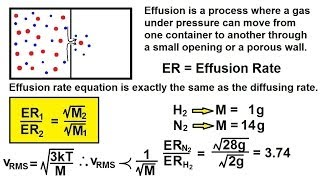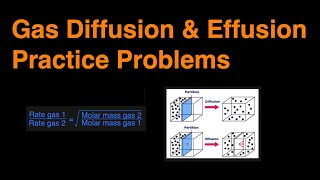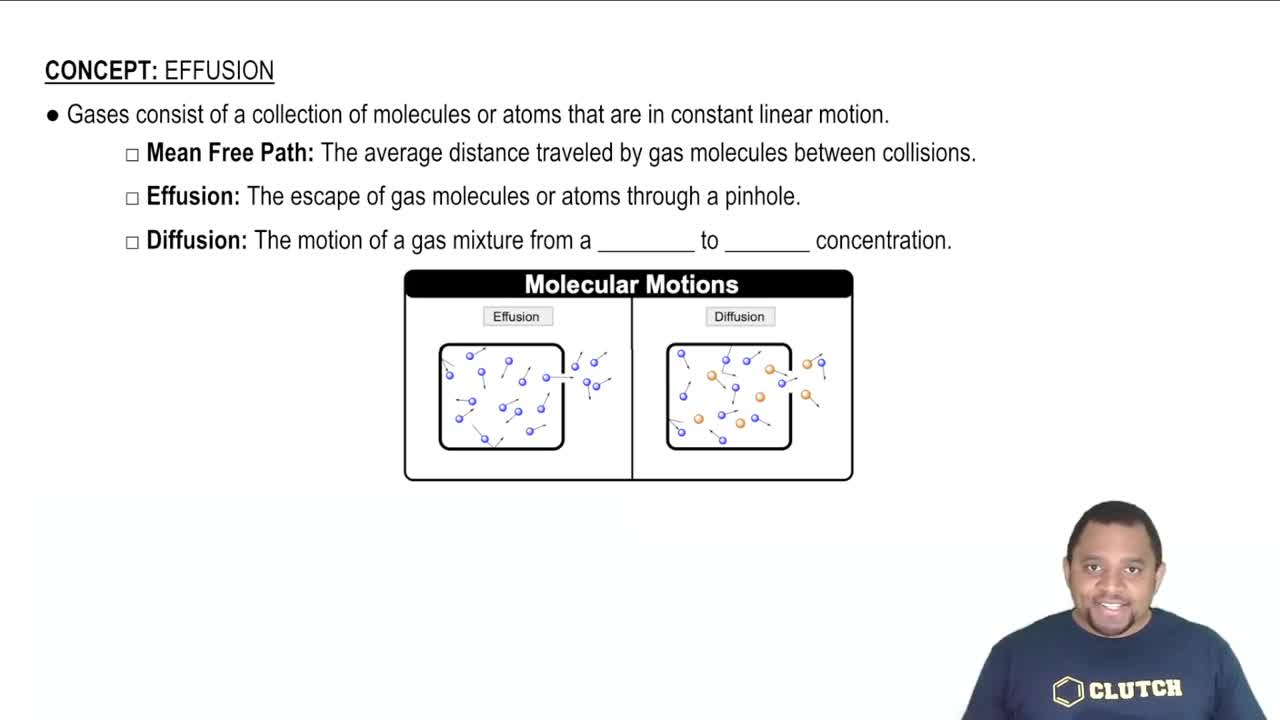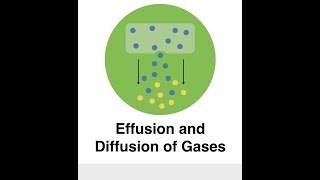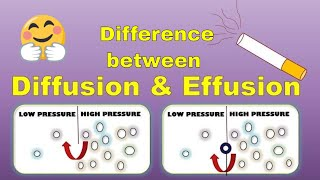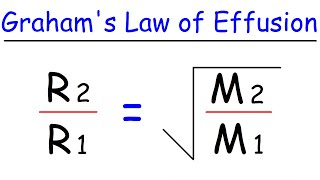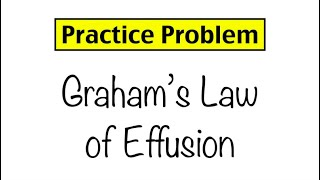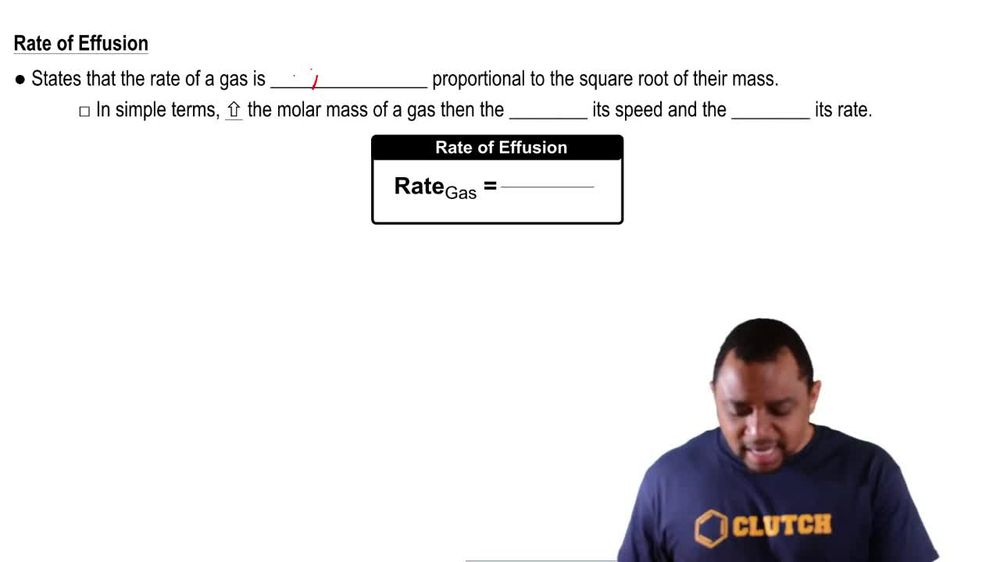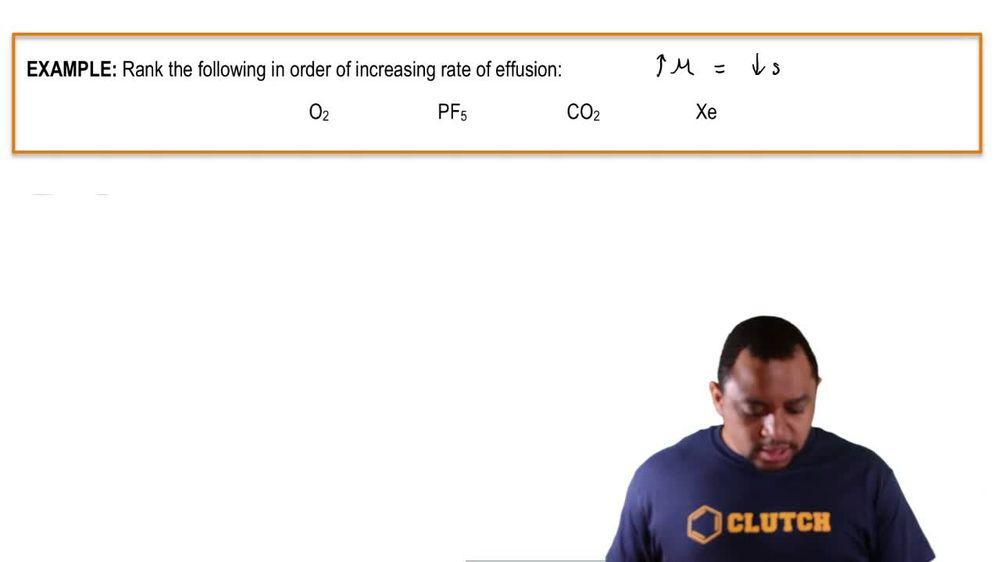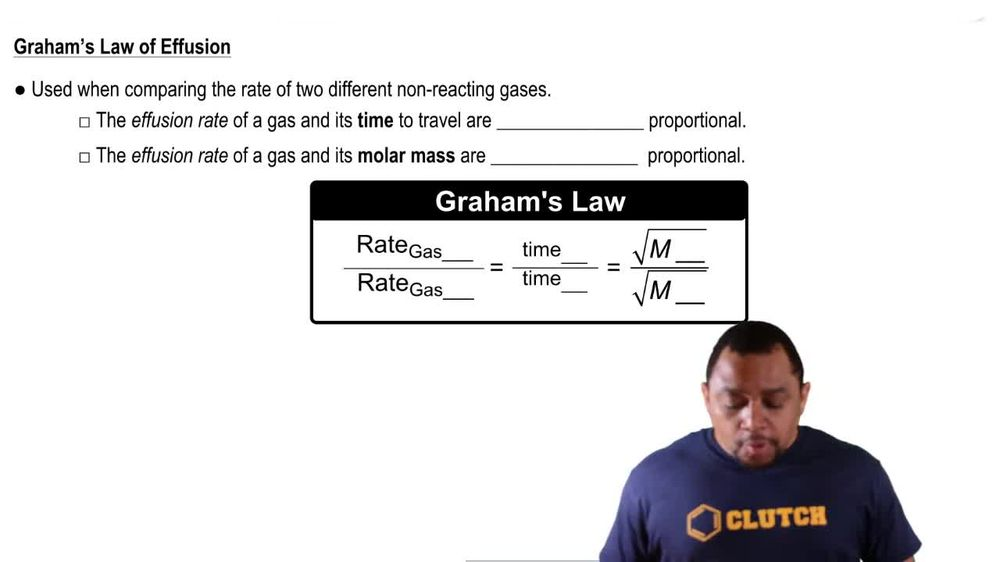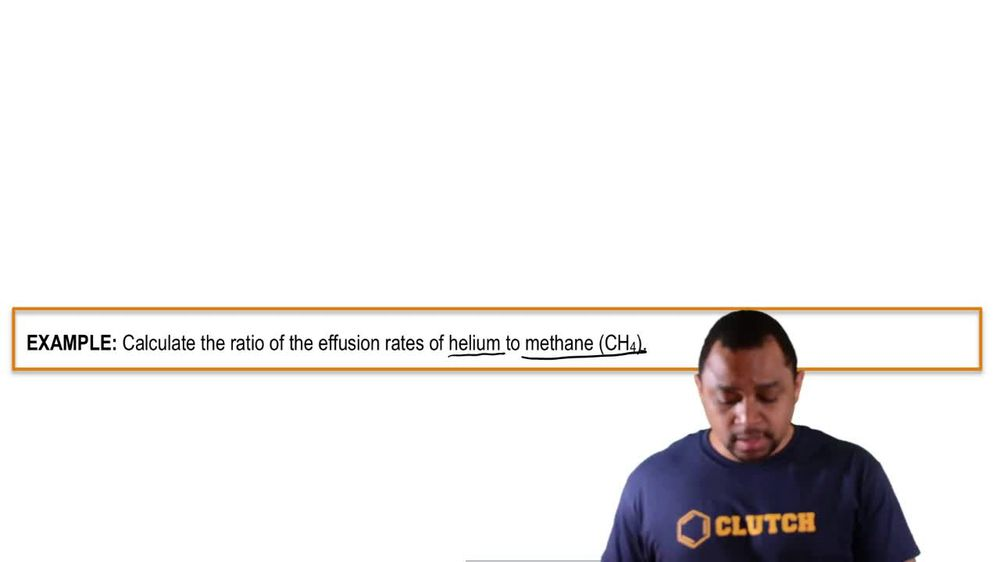Start typing, then use the up and down arrows to select an option from the list.
1. 7. Gases2. Effusion# Effusion

by Jules Bruno
93 views
0
the rate of a fusion states that the rate of a gas is inversely proportional to the square of their mass. So that means rate of gas equals one over our square root of Mueller, Mass. But what exactly does that mean? Well, in simple terms, it means increasing the molar mass of a gas means that we're going to lower its speed and the lower it's rate. So basically the Maura gas particle or molecule ways than the slower it's going to move. So that's basically the idea behind the rate of a fusion.